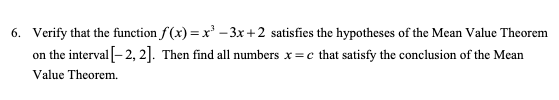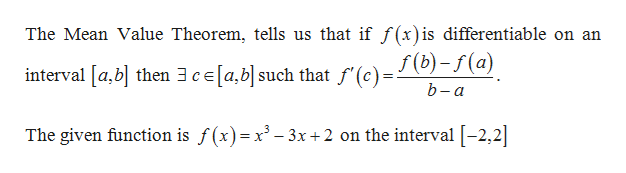# Verify that the function f(x)= x-3x+2 satisfies the hypotheses of the Mean Value Theorem6.on the interval-2, 2. Then find all numbers x=c that satisfy the conclusion of the MeanValue Theorem

Question
49 viewshelp_outlineImage TranscriptioncloseVerify that the function f(x)= x-3x+2 satisfies the hypotheses of the Mean Value Theorem 6. on the interval-2, 2. Then find all numbers x=c that satisfy the conclusion of the Mean Value Theorem fullscreen
check_circle

Step 1

Known fact:help_outlineImage TranscriptioncloseThe Mean Value Theorem, tells us that if f (x) is differentiable on an f (b)-f(a) interval [a,b then 3ce[a,b] such that f'(c) b-a The given function is f(x)=x3-3x +2 on the interval [-2,2 fullscreen
Step 2

Differentiate the funct...

### Want to see the full answer?

See Solution

#### Want to see this answer and more?

Solutions are written by subject experts who are available 24/7. Questions are typically answered within 1 hour.*

See Solution
*Response times may vary by subject and question.
Tagged in

### Calculus